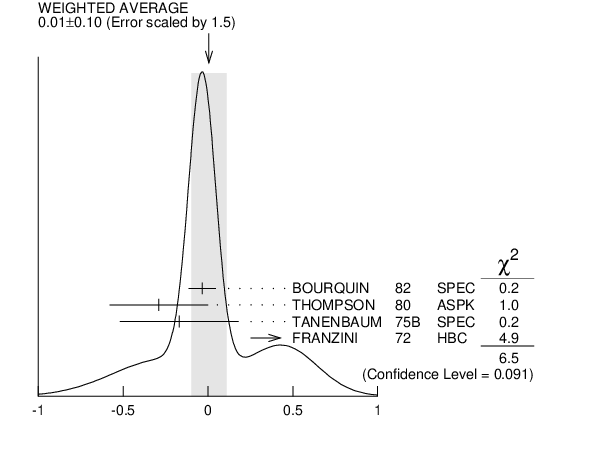# ${{\boldsymbol \Sigma}^{-}}$ DECAY PARAMETERS

See the Note on Baryon Decay Parameters'' in the neutron Listings. Older, outdated results have been omitted.

# ${\boldsymbol g}_{{{\boldsymbol V}}}/{\boldsymbol g}_{{{\boldsymbol A}}}$ FOR ${{\boldsymbol \Sigma}^{-}}$ $\rightarrow$ ${{\boldsymbol \Lambda}}{{\boldsymbol e}^{-}}{{\overline{\boldsymbol \nu}}_{{e}}}$ INSPIRE search

For the sign convention, see the Note on Baryon Decay Parameters'' in the neutron Listings. The value is predicted to be zero by conserved vector current theory. The values averaged assume CVC-SU(3) weak magnetism term.
VALUE EVTS DOCUMENT ID TECN  COMMENT
$\bf{ 0.01 \pm0.10}$ OUR AVERAGE  Error includes scale factor of 1.5.
$-0.034$ $\pm0.080$ 1620 1
 1982
SPEC SPS hyperon beam
$-0.29$ $\pm0.29$ 114
 1980
ASPK BNL hyperon beam
$-0.17$ $\pm0.35$ 55
 1975 B
SPEC BNL hyperon beam
$+0.45$ $\pm0.20$ 186 2, 1
 1972
HBC
1  The sign has been changed to agree with our convention.
2  The FRANZINI 1972 value includes the events of earlier papers.${\mathit g}_{{{\mathit V}}}/{\mathit g}_{{{\mathit A}}}$ for ${{\mathit \Sigma}^{-}}$ $\rightarrow$ ${{\mathit \Lambda}}{{\mathit e}^{-}}{{\overline{\mathit \nu}}_{{e}}}$
References:
 BOURQUIN 1982
ZPHY C12 307 Measurements of Hyperon Semileptonic Decays at the CERN Super Proton Synchrotron. 1. The ${{\mathit \Sigma}^{-}}$ $\rightarrow$ ${{\mathit \Lambda}}{{\mathit e}^{-}}{{\overline{\mathit \nu}}_{{e}}}$ Decay Mode
 THOMPSON 1980
PR D21 25 Studies in the BNL 21 ${\mathrm {GeV/}}\mathit c$ Negative Hyperon Beam. II. Leptonic Decays of ${{\mathit \Sigma}}$ and ${{\mathit \Xi}}$
 TANENBAUM 1975B
PR D12 1871 Leptonic Decays of the ${{\mathit \Sigma}^{-}}$ and ${{\mathit \Xi}^{-}}$ Hyperons
 FRANZINI 1972
PR D6 2417 Decay ${{\mathit \Sigma}^{\pm}}$ $\rightarrow$ ${{\mathit \Lambda}}{{\mathit e}^{\pm}}{{\mathit \nu}}$